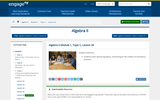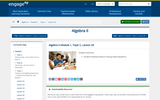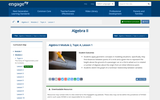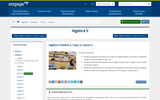Updating search results...

# 228 Results

View
Selected filters:
• Math 3Conditional Remix & Share Permitted
CC BY-NC-SA
Rating
0.0 stars
Overview:

Students will explore the equations of circles, their transformations, and derivation from the Pythagorean Theorem. Students will create circles on graph paper with specified center, radius, and containing 3 points. Students will determine the best location for a hospital to best serve the needs of Moore County.

Subject:
Math 3
Material Type:
Activity/Lab
Author:
William Allred
Carrie Robledo
05/19/2021Rating
3.66666666667 stars
Overview:

Sample Item provided by Smarter Balanced as preliminary examples of the types of items that students might encounter on the summative assessment. The item assesses whether the student has a solid understanding of writing equations to model a real-life situations and use the equations to find answers to questions within a context. MAT.HS.CR.2.0ACED.A.225

Subject:
Mathematics
Math 1
Math 3
Material Type:
Activity/Lab
Assessment
Provider:
Oregon Department of Education
Author:
Smarter Balanced Assessment Consortium
02/26/2019Rating
0.0 stars
Overview:

A Constructed Response Item provided by Smarter Balanced as preliminary examples of the types of items that students might encounter on the summative assessment. The item prompt students to produce a text or numerical response in order to collect evidence about their knowledge or understanding of representing constraints by equations and systems of equations, solving systems of linear equations and interpreting solutions as viable or nonviable options in a modeling context. MAT.HS.CR.2.0AREI.A.032

Subject:
Math 1
Math 2
Math 3
Material Type:
Activity/Lab
Assessment
Provider:
Oregon Department of Education
Author:
Smarter Balanced Assessment Consortium
02/26/2019Rating
4.0 stars
Overview:

A Constructed Response Item provided by Smarter Balanced as preliminary examples of the types of items that students might encounter on the summative assessment. The item prompt students to produce a text or numerical response in order to collect evidence about their knowledge or understanding of using structure of an expression to identify ways to rewrite it and rearrange formulas to highlight a specific quantity of interest. MAT.HS.CR.2.0ASSE.A.005

Subject:
Math 1
Math 3
Material Type:
Activity/Lab
Assessment
Provider:
Oregon Department of Education
Author:
Smarter Balanced Assessment Consortium
02/26/2019Rating
1.0 stars
Overview:

This is a classroom worksheet that has problems for adding and subtracting rational expressions.

Subject:
Math 3
Material Type:
Homework/Assignment
Provider:
Blackboard, Inc.
Author:
Jennifer Leahy
04/04/2018Conditional Remix & Share Permitted
CC BY-NC-SA
Rating
3.0 stars
Overview:

Students write explicit polynomial expressions for sequences by investigating successive differences of those sequences.

Subject:
Math 3
Material Type:
Lesson
Author:
EngageNY
02/22/2020Conditional Remix & Share Permitted
CC BY-NC-SA
Rating
0.0 stars
Overview:

Students explore the difference of two squares identity x2 − y2 = (x − y)(x + y) in the context of finding Pythagorean triples.

Subject:
Math 3
Material Type:
Lesson
Author:
EngageNY
02/22/2020Conditional Remix & Share Permitted
CC BY-NC-SA
Rating
0.0 stars
Overview:

Students find solutions to polynomial equations where the polynomial expression is not factored into linear factors.
Students construct a polynomial function that has a specified set of zeros with stated multiplicity.

Subject:
Math 3
Material Type:
Lesson
Author:
EngageNY
02/22/2020Conditional Remix & Share Permitted
CC BY-NC-SA
Rating
0.0 stars
Overview:

Students develop the distributive property for application to polynomial multiplication. Students connect multiplication of polynomials with multiplication of multi-digit integers.

Subject:
Math 3
Material Type:
Lesson
Author:
EngageNY
02/22/2020Conditional Remix & Share Permitted
CC BY-NC-SA
Rating
0.0 stars
Overview:

Students develop a division algorithm for polynomials by recognizing that division is the inverse operation of multiplication.

Subject:
Math 3
Material Type:
Lesson Plan
Author:
EngageNY
02/22/2020Conditional Remix & Share Permitted
CC BY-NC-SA
Rating
0.0 stars
Overview:

Students perform arithmetic operations on polynomials and write them in standard form.
Students understand the structure of polynomial expressions by quickly determining the first and last terms if the polynomial were to be written in standard form.

Subject:
Math 3
Material Type:
Lesson
Author:
EngageNY
02/22/2020Conditional Remix & Share Permitted
CC BY-NC-SA
Rating
0.0 stars
Overview:

Students work with polynomials with constant coefficients to prove polynomial identities.

Subject:
Math 3
Material Type:
Lesson
Author:
EngageNY
02/22/2020Conditional Remix & Share Permitted
CC BY-NC-SA
Rating
0.0 stars
Overview:

Students perform arithmetic by using polynomial identities to describe numerical relationships.

Subject:
Math 3
Material Type:
Lesson
Author:
EngageNY
02/22/2020Conditional Remix & Share Permitted
CC BY-NC-SA
Rating
0.0 stars
Overview:

Students apply polynomial identities to the detection of prime numbers.

Subject:
Math 3
Material Type:
Lesson
Author:
EngageNY
02/22/2020Conditional Remix & Share Permitted
CC BY-NC-SA
Rating
0.0 stars
Overview:

Students understand that the sum of two square roots (or two cube roots) is not equal to the square root (or cube root) of their sum.
Students convert expressions to simplest radical form.
Students understand that the product of conjugate radicals can be viewed as the difference of two squares.

Subject:
Math 3
Material Type:
Lesson
Author:
EngageNY
02/22/2020Conditional Remix & Share Permitted
CC BY-NC-SA
Rating
0.0 stars
Overview:

Students will factor certain forms of polynomial expressions by using the structure of the polynomials.

Subject:
Math 3
Material Type:
Lesson
Author:
EngageNY
02/22/2020Conditional Remix & Share Permitted
CC BY-NC-SA
Rating
0.0 stars
Overview:

Students will use the structure of polynomials to identify factors.

Subject:
Math 3
Material Type:
Lesson
Author:
EngageNY
02/22/2020Conditional Remix & Share Permitted
CC BY-NC-SA
Rating
0.0 stars
Overview:

Students will use the factored forms of polynomials to find zeros of a function.
Students will use the factored forms of polynomials to sketch the components of graphs between zeros.

Subject:
Math 3
Material Type:
Lesson
Author:
EngageNY
02/22/2020Conditional Remix & Share Permitted
CC BY-NC-SA
Rating
0.0 stars
Overview:

Students graph polynomial functions and describe end behavior based upon the degree of the polynomial.

Subject:
Math 3
Material Type:
Lesson
Author:
EngageNY
02/22/2020Conditional Remix & Share Permitted
CC BY-NC-SA
Rating
0.0 stars
Overview:

Students transition between verbal, numerical, algebraic, and graphical thinking in analyzing applied polynomial problems.

Subject:
Math 3
Material Type:
Lesson
Author:
EngageNY
02/22/2020Conditional Remix & Share Permitted
CC BY-NC-SA
Rating
0.0 stars
Overview:

Students transition between verbal, numerical, algebraic, and graphical thinking in analyzing applied polynomial problems.

Subject:
Math 3
Material Type:
Lesson
Author:
EngageNY
02/22/2020Conditional Remix & Share Permitted
CC BY-NC-SA
Rating
0.0 stars
Overview:

Students know and apply the Remainder Theorem and understand the role zeros play in the theorem.

Subject:
Math 3
Material Type:
Lesson
Author:
EngageNY
02/22/2020Conditional Remix & Share Permitted
CC BY-NC-SA
Rating
0.0 stars
Overview:

Students learn to fit polynomial functions to data values.

Subject:
Math 3
Material Type:
Lesson
Author:
EngageNY
02/22/2020Conditional Remix & Share Permitted
CC BY-NC-SA
Rating
0.0 stars
Overview:

Students model a cross-section of a riverbed with a polynomial function and estimate fluid flow with their algebraic model.

Subject:
Math 3
Material Type:
Lesson
Author:
EngageNY
02/22/2020Conditional Remix & Share Permitted
CC BY-NC-SA
Rating
0.0 stars
Overview:

Students define rational expressions and write them in equivalent forms.

Subject:
Math 3
Material Type:
Lesson
Author:
EngageNY
02/23/2020Conditional Remix & Share Permitted
CC BY-NC-SA
Rating
0.0 stars
Overview:

Students compare rational expressions by writing them in different but equivalent forms.

Subject:
Math 3
Material Type:
Lesson
Author:
EngageNY
02/23/2020Conditional Remix & Share Permitted
CC BY-NC-SA
Rating
0.0 stars
Overview:

Students multiply and divide rational expressions and simplify using equivalent expressions.

Subject:
Math 3
Material Type:
Lesson
Author:
EngageNY
02/23/2020Conditional Remix & Share Permitted
CC BY-NC-SA
Rating
0.0 stars
Overview:

Students solve rational equations, monitoring for the creation of extraneous solutions.

Subject:
Math 3
Material Type:
Lesson
Author:
EngageNY
02/23/2020Conditional Remix & Share Permitted
CC BY-NC-SA
Rating
0.0 stars
Overview:

Students solve word problems using models that involve rational expressions.

Subject:
Math 3
Material Type:
Lesson
Author:
EngageNY
02/23/2020Conditional Remix & Share Permitted
CC BY-NC-SA
Rating
0.0 stars
Overview:

Students solve simple radical equations and understand the possibility of extraneous solutions. They understand that care must be taken with the role of square roots so as to avoid apparent paradoxes.
Students explain and justify the steps taken in solving simple radical equations.

Subject:
Math 3
Material Type:
Lesson
Author:
EngageNY
02/23/2020Conditional Remix & Share Permitted
CC BY-NC-SA
Rating
0.0 stars
Overview:

Students develop facility in solving radical equations.

Subject:
Math 3
Material Type:
Lesson
Author:
EngageNY
02/23/2020Conditional Remix & Share Permitted
CC BY-NC-SA
Rating
0.0 stars
Overview:

Students define a complex number in the form a + bi, where a and b are real numbers and the imaginary unit i satisfies i 2 = −1. Students geometrically identify i as a multiplicand effecting a 90° counterclockwise rotation of the real number line. Students locate points corresponding to complex numbers in the complex plane.
Students understand complex numbers as a superset of the real numbers; i.e., a complex number a + bi is real when b = 0. Students learn that complex numbers share many similar properties of the real numbers: associative, commutative, distributive, addition/subtraction, multiplication, etc.

Subject:
Math 3
Material Type:
Lesson
Author:
EngageNY
02/23/2020Conditional Remix & Share Permitted
CC BY-NC-SA
Rating
0.0 stars
Overview:

Students understand the Fundamental Theorem of Algebra; that all polynomial expressions factor into linear terms in the realm of complex numbers. Consequences, in particular, for quadratic and cubic equations are understood.

Subject:
Math 2
Math 3
Material Type:
Lesson
Author:
EngageNY
02/23/2020Conditional Remix & Share Permitted
CC BY-NC-SA
Rating
0.0 stars
Overview:

Students apply geometric concepts in modeling situations. Specifically, they find distances between points of a circle and a given line to represent the height above the ground of a passenger car on a Ferris wheel as it is rotated a number of degrees about the origin from an initial reference point.
Students sketch the graph of a nonlinear relationship between variables

Subject:
Math 3
Material Type:
Lesson
Author:
EngageNY
02/23/2020Conditional Remix & Share Permitted
CC BY-NC-SA
Rating
0.0 stars
Overview:

Students observe identities from graphs of sine and cosine basic trigonometric identities and relate those identities to periodicity, even and odd properties, intercepts, end behavior, and the fact that cosine is a horizontal translation of sine.

Subject:
Math 3
Material Type:
Lesson
Author:
EngageNY
02/23/2020Conditional Remix & Share Permitted
CC BY-NC-SA
Rating
0.0 stars
Overview:

Students explore the historical context of trigonometry as motion of celestial bodies in a presumed circular arc.
Students describe the position of an object along a line of sight in the context of circular motion.
Students understand the naming of the quadrants and why counterclockwise motion is deemed the positive direction of turning in mathematics.

Subject:
Math 3
Material Type:
Lesson
Author:
EngageNY
02/23/2020Conditional Remix & Share Permitted
CC BY-NC-SA
Rating
0.0 stars
Overview:

Students define the tangent function and understand the historic reason for its name.
Students use special triangles to determine geometrically the values of the tangent function for 30°, 45°, and 60°.

Subject:
Math 3
Material Type:
Lesson
Author:
EngageNY
02/23/2020Conditional Remix & Share Permitted
CC BY-NC-SA
Rating
0.0 stars
Overview:

Students define the secant function and the co-functions in terms of points on the unit circle. They relate these names for these functions to the geometric relationships among lines, angles, and right triangles in a unit circle diagram.
Students use reciprocal relationships to relate the trigonometric functions and use these relationships to evaluate trigonometric functions for multiples of 30, 45, and 60 degrees.

Subject:
Math 3
Material Type:
Lesson
Author:
EngageNY
02/23/2020Conditional Remix & Share Permitted
CC BY-NC-SA
Rating
0.0 stars
Overview:

Students graph the sine and cosine functions and analyze the shape of these curves.
For the sine and cosine functions, students sketch graphs showing key features, which include intercepts; intervals where the function is increasing, decreasing, positive, or negative; relative maxima and minima; symmetries; end behavior; and periodicity.

Subject:
Math 3
Material Type:
Lesson
Author:
EngageNY
02/23/2020Conditional Remix & Share Permitted
CC BY-NC-SA
Rating
0.0 stars
Overview:

Students explore horizontal scalings of the graph of y =sin(x).
Students convert between degrees and radians.

Subject:
Math 3
Material Type:
Lesson
Author:
EngageNY